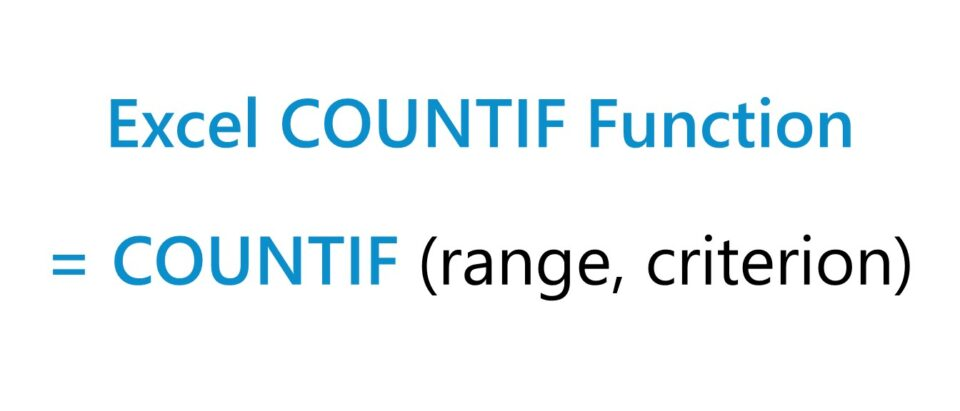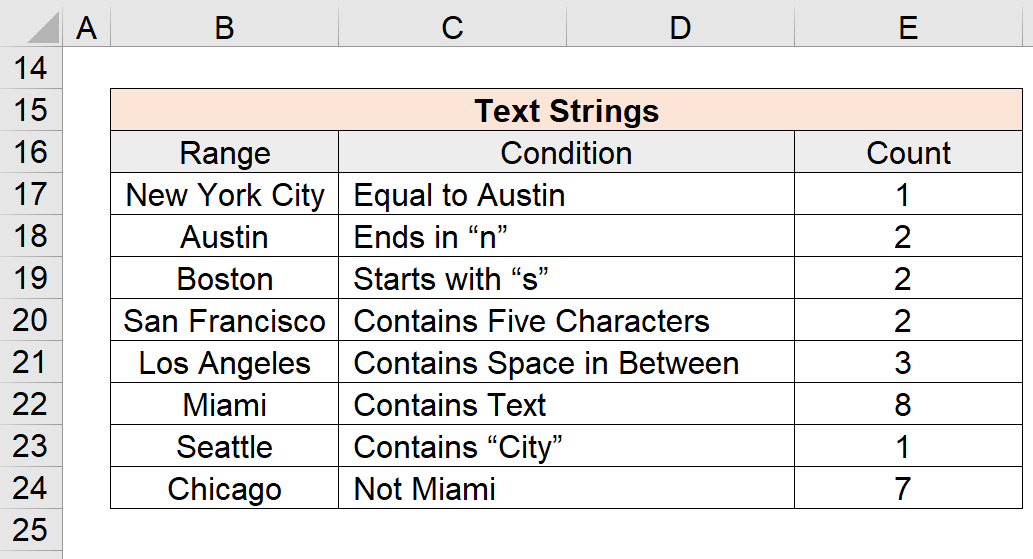Welcome to Wall Street Prep! Use code at checkout for 15% off.# COUNTIF Function

Guide to Understanding the "COUNTIF" Function in Excel## How to Use COUNTIF Function in Excel (Step-by-Step)

The Excel “COUNTIF” function is used to count the number of cells in a selected range that meets a specific condition.

Given one criterion, the COUNTIF function searches for an exact match to determine the total number of cells under which the condition is met.

For instance, the criteria could be related to finding the number of cells with values greater than, less than, or equal to a specific value.

The primary drawback to the “COUNTIF” function is that only one condition is supported. If the criteria in question consists of multiple conditions, the “COUNTIFS” function would be the more practical alternative.

In addition, the criterion is not case-sensitive, so the use of upper or lower case spelling in the text string does not affect the outcome.

## Excel COUNTIF Function Formula

The formula for using the COUNTIF function in Excel is as follows.

=COUNTIF(range, criterion)
• Range → The selected range containing the data set across which the function will search for the cells that match the stated criteria.
• Criterion → The specific condition that must be met in order for the function to count the cell.

## Numeric Criterion Syntax: Logical Operator

The range can contain text strings and numbers, whereas the criterion most often contains a logical operator such as:

Logical Operator Description
> Greater Than
< Less Than
= Equal To
>= Greater Than or Equal To
<= Less Than or Equal To
<> Not Equal To

## Text Strings, Date, Blank and Non-Blank Criterion

For text or date-based conditions, it is necessary to enclose the criterion in double quotes, otherwise the formula will not work.

Criterion Description
Text
• The criterion can also be related to containing certain text, such as the name of a city (e.g. “Boston”).
• There are exceptions to the necessity of the double quotes, however, such as for “True” or “False”.
Date
• The date criteria can count the entries that match a specific date (and must be wrapped in parentheses)
Blank Cells
• The (””) double quote (with nothing in between the quotes) can count the number of blank cells in the chosen range.
Non-Blank Cells
• The ”<>” operator can be used to count the number of non-blank cells
Cell References
• Cell references in the criteria should not be enclosed in quotes. For instance, the proper format if counting cells greater than cell B1 would be “>”&B1

## Wildcards in Criterion

The term “wildcards” refer to special characters such as a question mark, asterisk, or tilde.

Wildcard Description
(?)
• A question mark in the criteria will match any one character.
(*)
• An asterisk in the criteria will match zero (or more) characters of any sort, so any cells that contain a specific word.
• For example, “*th” will count any cell that ends in “th”, and “x*” will count cells that begin with “x”.
(~)
• A tilde matches a wildcard, e.g. “~?” would count any cells ending with a question mark.

## COUNTIF Function Calculator – Excel Model Template

We’ll now move on to a modeling exercise, which you can access by filling out the form below.Submitting...

## Part 1. Numeric Criteria COUNTIF Function Examples

Suppose we’re given the following range of numeric data to count the number of cells that meet various types of conditions.

The range is on the left column, while the condition is on the right column.

Range Condition
10 Equal to 10
12 Greater Than 10
15 Less Than 10
14 Greater Than or Equal to 10
6 Less Than or Equal to 10
8 Not Equal to 10
12 Blank Cells
10 Non-Blank Cells

The COUNTIF equations that we’ll use to count the matching cells are the following:

=COUNTIF(\$B\$6:\$B\$13,10) → Count = 2
=COUNTIF(\$B\$6:\$B\$13,”>10″) → Count = 4
=COUNTIF(\$B\$6:\$B\$13,”<10″) → Count = 2
=COUNTIF(\$B\$6:\$B\$13,”>=10″) → Count = 6
=COUNTIF(\$B\$6:\$B\$13,”<=10″) → Count = 4
=COUNTIF(\$B\$6:\$B\$13,”<>10″) → Count = 6
=COUNTIF(\$B\$6:\$B\$13,””) → Count = 0
=COUNTIF(\$B\$6:\$B\$13,”<>”) → Count = 8

## Part 2. Text Strings COUNTIF Function Examples

In the next section, we’ll work with the following data set of text strings, which are cities in this case.

Range Condition
New York City Equal to Austin
Austin Ends in “n”
Boston Starts with “s”
San Francisco Contains Five Characters
Los Angeles Contains Space in Between
Miami Contains Text
Seattle Contains “City”
Chicago Not Miami

The COUNTIF function equations that we’ll enter into Excel to count the cells that meet each of the corresponding criteria are the following:

=COUNTIF(\$B\$17:\$B\$24,”=Austin”) → Count = 1
=COUNTIF(\$B\$17:\$B\$24,”*n”) → Count = 2
=COUNTIF(\$B\$17:\$B\$24,”s*”) → Count = 2
=COUNTIF(\$B\$17:\$B\$24,”??????”) → Count = 2
=COUNTIF(\$B\$17:\$B\$24,”* *”) → Count = 3
=COUNTIF(\$B\$17:\$B\$24,”*”) → Count = 8
=COUNTIF(\$B\$17:\$B\$24,”City”) → Count = 1
=COUNTIF(\$B\$17:\$B\$24,”<>Miami”) → Count = 7Turbo-charge your time in Excel Used at top investment banks, Wall Street Prep's Excel Crash Course will turn you into an advanced Power User and set you apart from your peers.